# Chamfer function for fun

edited July 14

Hello,

Some EDA tool has chamfer function, which can add extra pattern to prevent
metal trace from transition with 90 degree angle or less ( Maybe not often to see in VLSI ?)
Someone told me, it can be done by " round corner " function, but I tried and failed, due to
triangle compensation is preferred.. Share below code for fun, any smart method or comment will be appreciated.

BTW, the initial length of chamfer ( tear_drop ) is 20 " um "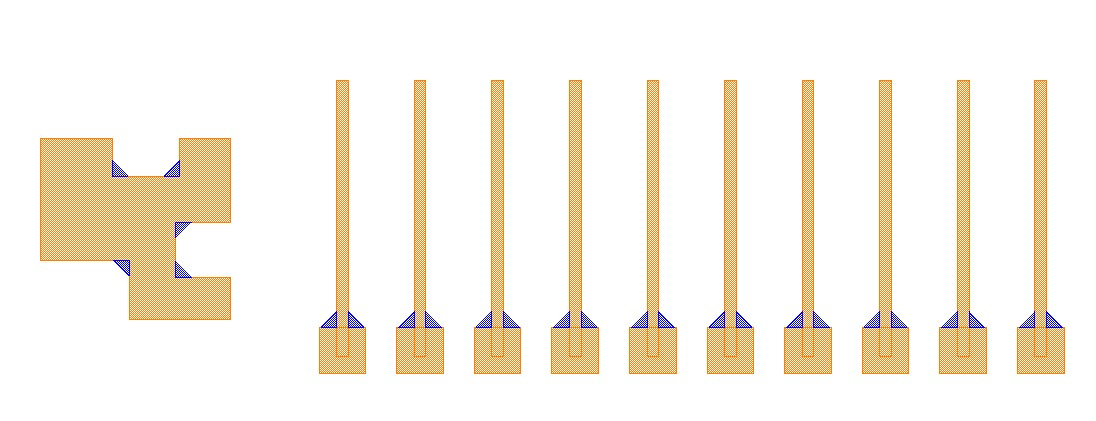``````import pya

cellview = pya.CellView.active()
layout = cellview.layout()
top = cellview.cell

metal = layout.layer(9 , 0)
tear_drop = layout.layer(6 , 0)

target_90 = pya.Region( layout.top_cell().begin_shapes_rec(metal)).with_angle(270, 360 , False)
count_90 = target_90.size()
first_edge_iter_90 = target_90.first_edges().each()
second_edge_iter_90 = target_90.second_edges().each()
target_90_pairs_set = [ k for k in target_90.each() ]
center_90_set = []
start_point_set = []
end_point_set = []

def center_90():
for i in range(0, count_90):
center = target_90_pairs_set[i].first.crossing_point(target_90_pairs_set[i].second)
center_90_set.append(center)

def start_point():
for j in range(0, count_90):
P1 = target_90_pairs_set[j].first.d().x
P2 = target_90_pairs_set[j].first.d().y
P3 = target_90_pairs_set[j].first.length()
P4 = center_90_set[j].x
P5 = center_90_set[j].y
length = 20000

if P1 >= 0 and P2 == 0 and P3 >= length:
start_point_set.append(pya.Point(P4 - length, P5))
elif P1 >= 0 and P2 == 0 and P3 < length:
start_point_set.append(pya.Point(P4 - P3, P5))
elif P1 < 0 and P2 == 0 and P3 >= length:
start_point_set.append(pya.Point(P4 + length, P5))
elif P1 < 0 and P2 == 0 and P3 < length:
start_point_set.append(pya.Point(P4 + P3, P5))
elif P1 == 0 and P2 >= 0 and P3 >= length:
start_point_set.append(pya.Point(P4 , P5 -length))
elif P1 == 0 and P2 >= 0 and P3 < length:
start_point_set.append(pya.Point(P4 , P5 - P3))
elif P1 == 0 and P2 < 0 and P3 >= length:
start_point_set.append(pya.Point(P4 , P5 + length))
elif P1 == 0 and P2 < 0 and P3 < length:
start_point_set.append(pya.Point(P4 , P5 + P3))
else:
pass

def end_point():
for j in range(0, count_90):
P1 = target_90_pairs_set[j].second.d().x
P2 = target_90_pairs_set[j].second.d().y
P3 = target_90_pairs_set[j].second.length()
P4 = center_90_set[j].x
P5 = center_90_set[j].y
length = 20000

if P1 >= 0 and P2 == 0 and P3 >= length:
end_point_set.append(pya.Point(P4 + length, P5))
elif P1 >= 0 and P2 == 0 and P3 < length:
end_point_set.append(pya.Point(P4 + P3, P5))
elif P1 < 0 and P2 == 0 and P3 >= length:
end_point_set.append(pya.Point(P4 - length, P5))
elif P1 < 0 and P2 == 0 and P3 < length:
end_point_set.append(pya.Point(P4 - P3, P5))
elif P1 == 0 and P2 >= 0 and P3 >= length:
end_point_set.append(pya.Point(P4 , P5 + length))
elif P1 == 0 and P2 >= 0 and P3 < length:
end_point_set.append(pya.Point(P4 , P5 + P3))
elif P1 == 0 and P2 < 0 and P3 >= length:
end_point_set.append(pya.Point(P4 , P5 - length))
elif P1 == 0 and P2 < 0 and P3 < length:
end_point_set.append(pya.Point(P4 , P5 - P3))
else:
pass

def tear():
for x in range(0, count_90):
P1 = start_point_set[x]
P2 = center_90_set[x]
P3 = end_point_set[x]
poly = pya.Polygon( [ P1, P2, P3 ] )
top.shapes(tear_drop).insert(poly)

center_90()
start_point()
end_point()
tear()
``````

• edited July 15

Now you are challenging meHere is a simple solution that uses a DRC function with an intra-polygon space check with an above-90 angle limit to generate notch violation markers on inner 90 degree-corners. 1µm is the edge length of the marker:

``````original = input(...)
patches = original.notch(1.um, angle_limit(91.0))
(original + patches.polygons).output(...)
``````

Result: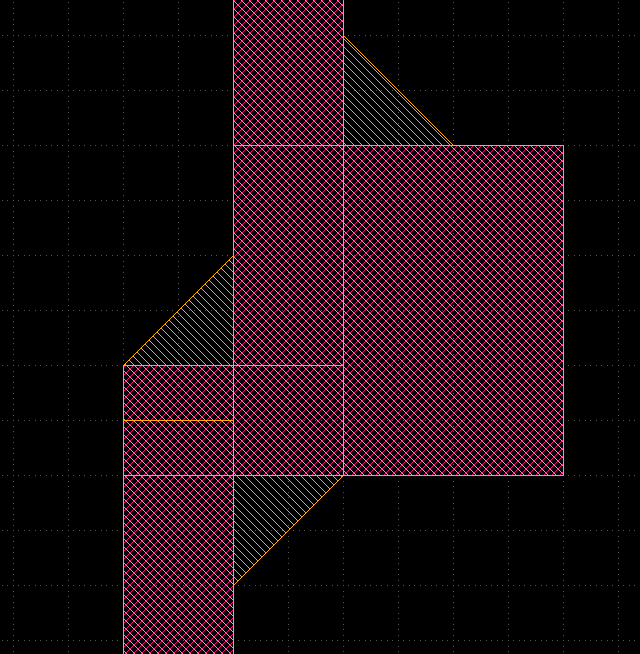This approach will not work properly on edges less than 1µm length because then the chamfering gets non-45 degree.

The rounded-corner approach works as well (DRC) when you set the outer corner radius to 0 and use the octagon approximation (n=8):

``````original = input(...)
f = 1.0 + Math::sqrt(0.5)
original.rounded_corners(f * 1.um, 0.um, 8).output(...)
``````

Caveat: the corner rounding does not apply full radius on edges shorter than f µm (f is 1.707...) but applies a smaller chamfering: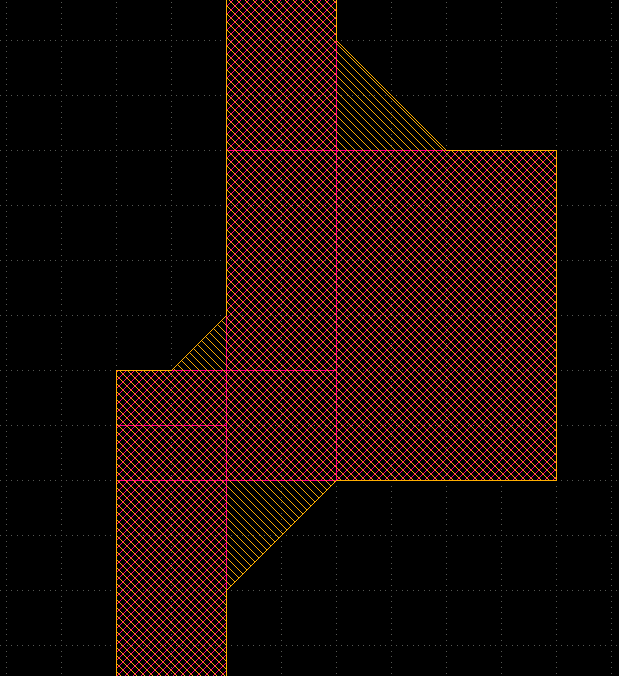So both approaches have a slight disadvantage that you may be able to mitigate with specific design rules.

Matthias

• hello Matthias,

You should be kidding me..Method one already works perfectly to me, I have no ruby background, so I missed many great information in the manual, even can not understand properly.You solution always hit the problem in 3 rows, it is magic.

I am not sure why I can not upload a gds file on.. so please see below image:
the solution also can make the tear for a trace connect to circle, it's already good enough to tape out mask if merged polygons, even better than what Cadence did, but I will keep trying to make it as " triangle " shape to reduce file size if convert to MEBES. Maybe will share another long code again, when it's done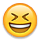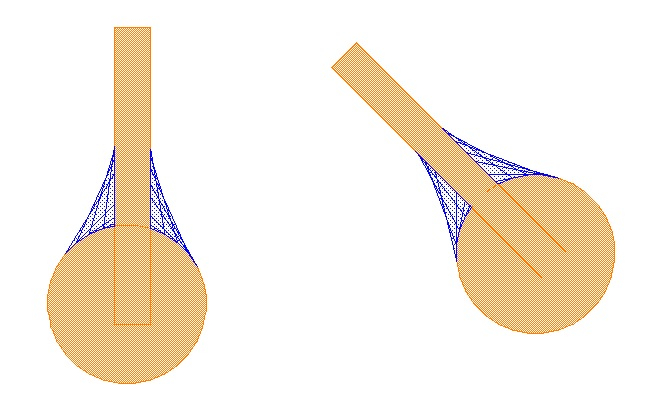• Very good, thanks for the feedbackYou can upload GDS if you zip it. This forum does now know about our favourite file formats ...

Matthias

• edited July 17

Hello Matthias,

It's my bad, I should notice that, updated test gds ok, and trying to solve it with math.(sin , cos, tan) friends..

• edited July 18

Hello Matthias,

Try & error, come out below code, it seems work,
Thanks for your perfect reply and this creative toolVincent

``````from pya import *
import math

cellview = pya.CellView.active()
layout = cellview.layout()
top = cellview.cell

metal = layout.layer(9 , 0)
bump = layout.layer(6 , 0)
tear_drop = layout.layer(5,0)

#############################################################

tear_length = 30000

#############################################################

pillar = pya.Region(top.begin_shapes_rec(bump))
metal_poly = pya.Region(top.begin_shapes_rec(metal))
dummy = ( pillar + metal_poly).merged()
#dummy = ( pillar + (pillar.sized(50000) & metal_poly)).merged()  # use this dummy instead of previous will enhance success rate

target = dummy.with_angle(181, 359 , False)
target_pairs = [ k for k in target.each() ]

for pair in target_pairs:
if pair.first.length() > pair.second.length():
P1 = pair.first.p2 + tear_length*(pair.first.p1 - pair.first.p2)/pair.first.length()
P2 = pair.first.p2
P3 = pair.second.p1 + tear_length*(pair.second.p2 - pair.second.p1)/pair.second.length()
pillar_hull_coordinate = []
pillar_corner_to_p3 = []
for k in [ k for k in pillar ]:
for l in range(k.num_points_hull()):
pillar_hull_coordinate.append(k.point_hull(l))
pillar_corner_to_p3.append(k.point_hull(l).distance(P3))
for k in pillar_hull_coordinate:
if k.distance(P3) == min(pillar_corner_to_p3):
P3 = k
tear = pya.Polygon([ P1, P2, P3 ])
top.shapes(tear_drop).insert(tear)

else:
pass

if pair.first.length() < pair.second.length():
P1 = pair.first.p2 + tear_length*(pair.first.p1 - pair.first.p2)/pair.first.length()
P2 = pair.first.p2
P3 = pair.second.p1 + tear_length*(pair.second.p2 - pair.second.p1)/pair.second.length()
pillar_hull_coordinate = []
pillar_corner_to_p1 = []
for k in [ k for k in pillar ]:
for l in range(k.num_points_hull()):
pillar_hull_coordinate.append(k.point_hull(l))
pillar_corner_to_p1.append(k.point_hull(l).distance(P1))
for k in pillar_hull_coordinate:
if k.distance(P1) == min(pillar_corner_to_p1):
P1 = k
tear = pya.Polygon([ P1, P2, P3 ])
top.shapes(tear_drop).insert(tear)
``````
• edited July 20

Of course this solution is valid too and I really want encourage users to try their own ways. So please go ahead and thanks for sharing the code!There are a few things you're probably aware of:

• It's slower because of the Python loop
• You will see all patches cells on top level of the hierarchy. The DRC-based solutions can be made hierarchy-aware (patches pushed down into the hierarchy) by simply using "deep" mode.

Kind regards,

Matthias